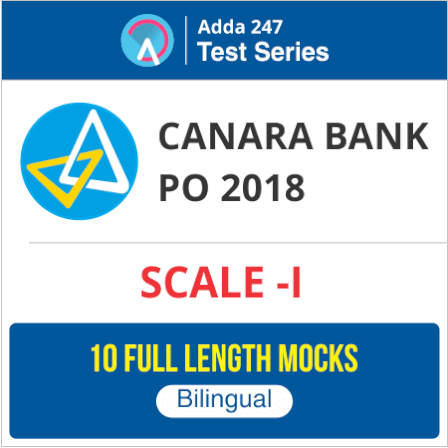# Reasoning Quiz for IBPS Clerk Prelims: 2nd December

###Reasoning Questions for IBPS Clerk 2018

Reasoning Ability is an onerous section. With the increasing complexity of questions, it becomes hard for one to give it the cold shoulder. The only way to make the grade in this particular section in the forthcoming banking exams like IBPS Clerk Prelims is to practice continuously with all your heart and soul. And, to let you practice with the best of the latest pattern questions, here is the Adda247 Reasoning Quiz based on the exact same pattern of questions that are being asked in the exams.

Directions (1-5): Study the following information carefully and answer the given questions.

Eight friends i.e. J, K, P, Z, C, B, D, and G have decided to do the lunch in a restaurant. They have seated on two parallel rows, each having five seats. Each row has one vacant seat but those vacant seats are not opposite to each other. P, C, Z and D sit in row 1 and face South, whenever J, K , G and B sit in row 2 and faces North. They like different colors i.e. Blue, Green, Grey, Black, Red, Yellow, White and Pink, but not necessary in same order.
The one who likes Grey sits 2nd right of G but both do not sit at any extreme end. The one who faces the one, who likes Grey, sits immediate left of the vacant seat of row 1. There is only one person sits between vacant seat of row 2 and J. The one who faces the vacant seat of row 2 is C who likes Yellow. P sits 3rd right of C. The one who likes Blue is immediate neighbor of the one who faces P. The one who likes green faces the one who likes Blue. K does not like Blue. Z does not like Green. The one who likes white faces vacant seat of row 1. The one, who likes Pink sits second to the right of the one who likes Red.
Q1. Who among the following likes White?
(a) B
(b) K
(c) Z
(d) C
(e) J
Q2. Who among the following sits 2nd left of the one who likes Green?
(a) B
(b) K
(c) Z
(d) No one
(e) P
Q3. Who among the following likes Black?
(a) C
(b) D
(c) P
(d) B
(e) G
Q4. Who among the following faces the one who likes Red?
(a) B
(b) K
(c) Z
(d) C
(e) P
Q5. How many person sit between G and J?
(a)Two
(b) One
(c) Four
(d) Three
(e) None
Directions (6-10): Answer the following questions referring to the symbol-letter-number sequence given below:
X W 5 L S 2 & Z%O M 1 N 3 G B I 9 D 6 Y 4 ~ J 7 E
Q6. Which of the following is exactly midway between the tenth element from the right end and fifth element from the left end?
(a) O
(b) M
(c) 1
(d) %
(e) None of these
Q7. How many letters are there in the above sequence which are immediately preceded by a number and immediately followed by a consonant?
(a) None
(b) one
(c) Two
(d) Three
(e) None of these
Q8.  What should come in place of the question mark (?) in the following sequence?
?,  2OL,  %N&
(a) 5&X
(b) LZB
(c) SZ5
(d) 5&N
(e) None of these
Q9. Which of the following is the eleventh element to the right of the second element from the left end in the above sequence?
(a) 9
(b) 1
(c) Y
(d) 4
(e) None of these
Q10. If the whole series is written in reverse order (E at the leftmost end and X at the rightmost end), then which of the following element will be second to the right of W?
(a) &
(b) X
(c) 5
(d) L
(e) None
Directions (11-15):  In these questions, relationships between elements is shown in the statements. These statements are followed by two conclusions. Give answer
Q11.  Statements: G≤F=L≤J; J≤K=H
Conclusions:     I. H=G     II. G<H
(a) If only conclusion I follows.
(b) If only conclusion II follows.
(c) If either conclusion I or conclusion II follows.
(d) If neither conclusion I nor conclusion II follows.
(e) If both conclusions I and II follow.
Q12.  Statements: P<R<S<T>U
Conclusions:     I. U<R     II. T>P
(a) If only conclusion I follows.
(b) If only conclusion II follows.
(c) If either conclusion I or conclusion II follows.
(d) If neither conclusion I nor conclusion II follows.
(e) If both conclusions I and II follow.
Q13.  Statements: T>U≥V≥W; X<Y=W>Z
Conclusions:     I. Z>U     II. W<T
(a) If only conclusion I follows.
(b) If only conclusion II follows.
(c) If either conclusion I or conclusion II follows.
(d) If neither conclusion I nor conclusion II follows.
(e) If both conclusions I and II follow.
Q14.  Statements: K<L<M<N; M<O<P
Conclusions:     I. P>K     II. N>O
(a) If only conclusion I follows.
(b) If only conclusion II follows.
(c) If either conclusion I or conclusion II follows.
(d) If neither conclusion I nor conclusion II follows.
(e) If both conclusions I and II follow.
Q15.Statements: B<A<C; A>D≤E
Conclusions:   I. B≤E     II. C>E
(a) If only conclusion I follows.
(b) If only conclusion II follows.
(c) If either conclusion I or conclusion II follows.
(d) If neither conclusion I nor conclusion II follows.
(e) If both conclusions I and II follow.

You may also like to Read: